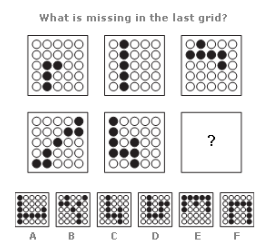1.A. A B. B C. C D. D

Explanation: :

The number of black dots in each grid increases by 1 each time, starting with the top left grid and working to the right, top row then bottom row.

2.A. Q B. R C. H D. A

Explanation: :

Adding the three numbers in each square together gives the numerical value of the letter at the centre of each square.

3.A. 15 B. 12 C. 9 D. 6

Explanation: :

The value at each corner of the diagram equals the difference between the sums of the numerical values of the letters in the boxes adjacent to the corner.

4.A. G B. E C. K D. V

Explanation: :

Convert each letter to its numerical value, and read each pair of values as 2 digit numbers.

In each row, the number in the centre equals the difference between the 2 digit values on the left and right.

5.A. Y B. H C. C D. E